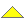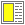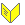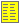Test of Object-oriented Programs - slide 12 : 35

Cyclomatic Complexity - Metric and Test Cases
Calculation of the test metric and finding test cases
• The cyclomatic complexity of the program unit P is determined

• Can be done the flow graph - by use of simple graph theory concepts

• The number of regions of the flow graph   or

• The number of predicate notes + 1

• Construct a test case for each independent path

• The test case should follow the control-flow of the path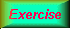Cyclomatic complexity of GCD